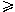## Calculation of the thermodynamic properties of aqueous species at high pressures and temperatures. Effective electrostatic radii, dissociation constants and standard partial molal properties to 1000 °C and 5 kbar

### Abstract

Within the framework of the revised HKF (H. C. Helgeson, D. H. Kirkham and G. C. Flowers, Am. J. Sci., 1981, 281, 1249) equations of state (J. C. Tanger IV and H. C. Helgeson, Am. J. Sci., 1988, 288, 19), prediction of the standard partial molal thermodynamic properties of aqueous ions and electrolytes at high pressures and temperatures requires values of the effective electrostatic radii of the ions (re), as well as provision for the temperature and pressure dependence of the relative permittivity of the solvent, H2O. Values of the relative permittivity of H2O, together with the Born functions needed to compute the standard partial molal Gibbs free energy, enthalpy, entropy, heat capacity and volume of solvation were calculated as a function of temperature and density from a modified version of the Uematsu–Franck equation (M. Uematsu and E. U. Franck, J. Phys. Chem. Ref. Data, 1980, 9, 1291). The temperature/pressure dependence of re is described in terms of a solvent function designated by g, which was evaluated in the present study at temperatures and pressures to 1000 °C and 5 kbar by regressing experimental standard partial molal heat capacities and volumes of NaCl reported in the literature together with published dissociation constants for NaClo at supercritical temperatures and pressures using the revised HKF equations of state for aqueous species. The calculated values of re decrease substantially with increasing temperature at constant pressure ⩽2 kbar, and with decreasing pressure at constant temperature400 °C. The equations and parameters summarized below permit calculation of the standard partial molal properties of aqueous species from the revised HKF equations of state over a much more extensive range of temperature than was previously possible.

## Article information

Article type
Paper

J. Chem. Soc., Faraday Trans., 1992,88, 803-826

### Permissions### Calculation of the thermodynamic properties of aqueous species at high pressures and temperatures. Effective electrostatic radii, dissociation constants and standard partial molal properties to 1000 °C and 5 kbar

E. L. Shock, E. H. Oelkers, J. W. Johnson, D. A. Sverjensky and H. C. Helgeson, J. Chem. Soc., Faraday Trans., 1992, 88, 803 DOI: 10.1039/FT9928800803